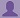SHARE
HELP

To start with, Sr2Jr’s first step is to reduce the expenses related to education. To achieve this goal Sr2Jr organized the textbook’s question and answers. Sr2Jr is community based and need your support to fill the question and answers. The question and answers posted will be available free of cost to all.# Absolute C++

Authors:
Walter Savitch ,kenrick Mock
Exercise:
Programming Projects
Chapter:
Flow Of Control
Edition:
5
ISBN:
9780132846813
Question:
1
 Previous Next

###Question

It is difficult to make a budget that spans several years, because prices are not stable. If your company needs 200 pencils per year, you cannot simply use this year’s price as the cost of pencils two years from now. Because of inflation the cost is likely to be higher than it is today. Write a program to gauge the expected cost of an item in a specified number of years. The program asks for the cost of the item, the number of years from now that the item will be purchased, and the rate of inflation. The program then outputs the estimated cost of the item after the specified period. Have the user enter the inflation rate as a percentage, such as 5.6 (percent). Your program should then convert the percentage to a decimal fraction, such as 0.056, and should use a loop to estimate the price adjusted for inflation. ( Hint: Use a loop.)

###Answer

``````#include <iostream>
using namespace std;

int main()
{
int nYears, i;
double cost, rate;
cout.precision(3);

cout << "Enter the number of years in which the item will be purchased: ";
cin >> nYears;
cout << "Enter the cost of the item: \$";
cin >> cost;
cout << "Enter the inflation rate as a percentage: ";
cin >> rate;

rate = rate / 100;

for(i=0;i<nYears;i++)
cost = cost + cost*rate;

cout << "The cost of the item after " << nYears << " years will be \$ " << cost << ".";

return 0;
} ``````

### Discussions

Post the discussion to improve the above solution.